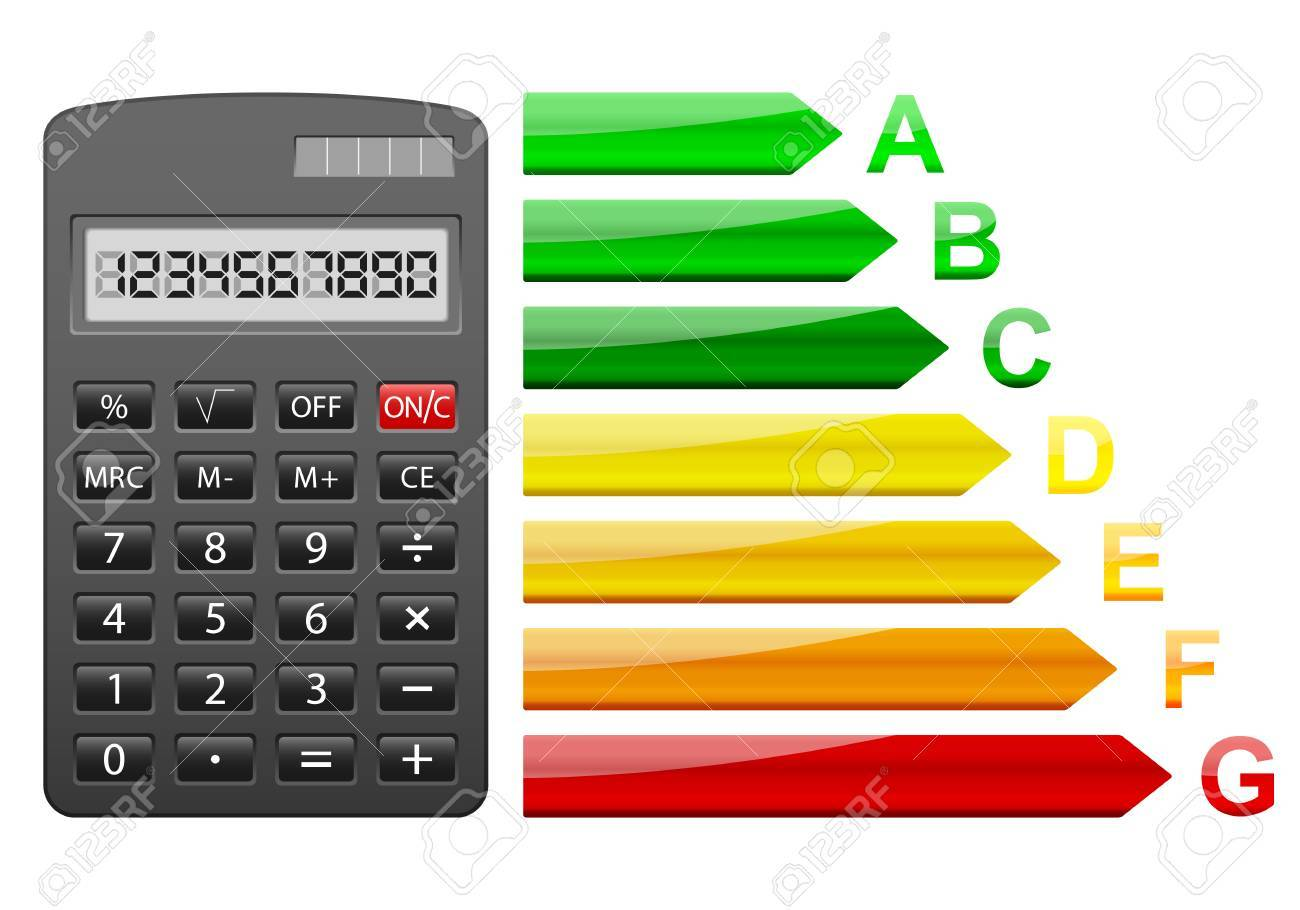## How can Calculators be More Efficient to Users?Studying mathematics for the sake of mathematics, formulation of conjectures to model real life situations and indeed the application of mathematical knowledge has helped to improve insight about nature.

The advances in pure mathematics, natural sciences, engineering, medicine, finance and the social sciences has lead to the formulation of more practical problems requiring practical solutions. Some of these needed solutions however pose a great strain on the human numerical capacity, hence the need for calculators.

Antiquity supplied itself with a means to eliminate this strain on the human numerical capacity and indeed eliminate error in calculations. The first calculators were recognized as mere counting materials and devices; they were stones, pebbles, bones and the abacus.

It was not until the 17th century that the term calculating machine or mechanical calculator became widespread. Wilhelm Schickard built the earliest modern attempt at a mechanical calculator. His mechanical calculator comprised Abacus made of Napier bones which performed multiplication and division operations and a dialed pedometer which performed addition and subtraction operations. However, he was not very successful.